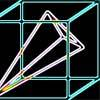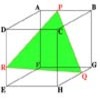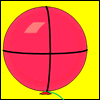Search by Topic

Resources tagged with Coordinates - 3D similar to Cheese Cutting:

Filter by: Content type:
Age range:
Challenge level:

There are 7 results

Broad Topics > Coordinates and Coordinate Geometry > Coordinates - 3D3D Treasure Hunt

Age 14 to 18 Challenge Level:

Some treasure has been hidden in a three-dimensional grid! Can you work out a strategy to find it as efficiently as possible?Four Points on a Cube

Age 16 to 18 Challenge Level:

What is the surface area of the tetrahedron with one vertex at O the vertex of a unit cube and the other vertices at the centres of the faces of the cube not containing O?Plane to See

Age 16 to 18 Challenge Level:

P is the midpoint of an edge of a cube and Q divides another edge in the ratio 1 to 4. Find the ratio of the volumes of the two pieces of the cube cut by a plane through PQ and a vertex.Cubestick

Age 16 to 18 Challenge Level:

Stick some cubes together to make a cuboid. Find two of the angles by as many different methods as you can devise.Cut Cube

Age 16 to 18 Challenge Level:

Find the shape and symmetries of the two pieces of this cut cube.Mesh

Age 16 to 18 Challenge Level:

A spherical balloon lies inside a wire frame. How much do you need to deflate it to remove it from the frame if it remains a sphere?Flight Path

Age 16 to 18 Challenge Level:

Use simple trigonometry to calculate the distance along the flight path from London to Sydney.